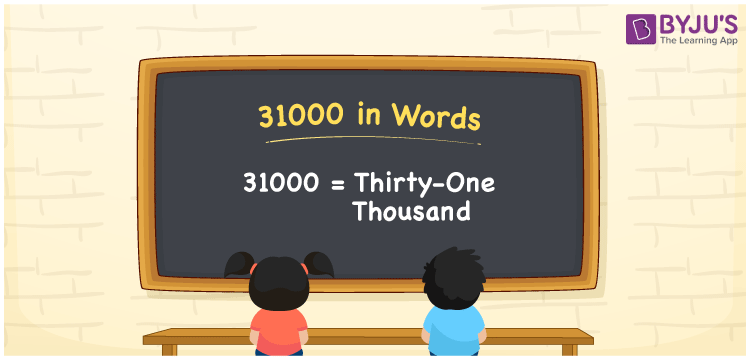# 31000 in Words

31000 in words is represented as Thirty-One Thousand. That is the spelling of 31000 in English is Thirty-One Thousand. If you have purchased golden bangles worth Rs. 31000, then you can express it as “I have purchased golden bangles worth Rs. 31000”. The number 31000 is a cardinal number or a counting number. To check any number in words, we have to identify the place values of each digit. In this article, let us learn how to write the number 31000 in words.

 31000 in words Thirty-One Thousand Thirty-One Thousand in numerical form 31000

## 31000 in English Words

Generally we use the English alphabet to write the numbers in words. Thus, we can express 31000 in English as “Thirty-One Thousand”.## How to Write 31000 in Words?

Place value chart is essential to write any number in words. Place value denotes the position of a digit in a number. Hence, we can write the number 31000 in words with the help of the place value method.

 Ten thousands Thousands Hundreds Tens Ones 3 1 0 0 0

Hence, we can write the expanded form as:

3 x Ten thousand + 1 x Thousand + 0 x Hundred + 0 × Ten + 0 × One

= 3 x 10000 + 1 x 1000 + 0 x 100 + 0 x 10 + 0 x 1

= 30000 + 1000

= 31000

= Thirty-One Thousand

Therefore, 31000 in words is written as Thirty-One Thousand.

Interesting way of writing 31000 in words

3 = Three

31 = Thirty-one

310 = Three hundred and ten

3100 = Three thousand one hundred

31000 = Thirty-One Thousand

Thus, the word form of the number 31000 is Thirty-One Thousand.

31000 is a natural number whose successor is 31001 and the predecessor is 30999.

• 31000 in words – Thirty-One Thousand
• Is 31000 an odd number? – No
• Is 31000 an even number? – Yes
• Is 31000 a perfect square number? – No
• Is 31000 a perfect cube number? – No
• Is 31000 a prime number? – No
• Is 31000 a composite number? – Yes

## Frequently Asked Questions on 31000 in Words

Q1

### How to write 31000 in words?

31000 in words is written as Thirty-One Thousand.
Q2

### What is the value of 31000 minus 26500?

31000 – 26500 = 4500 Therefore, the value of 31000 minus 26500 is 4500.
Q3

### 31000 is a prime number. True or False.

False, 31000 is not a prime number.